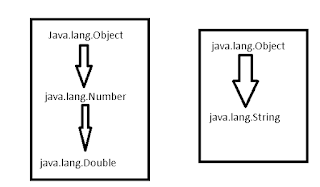# How to convert String to Double or Double to String in java | Double example

### Double String exampleThe string is a sequence of characters enclosed in double quotes.

Double is numeric data type of double precision floating values which hold double primitive types. Both have different values for a different purpose. Sometimes, In our applications, It is required to convert a string to double or vice-versa in java. This is a basic common requirement for knowing developers. Double holds 64 bits numbers, double value has d or D added to its value. d or D is not compulsory.

#### Example

``````String s=new String("123.45");
String s1="23.45";
Double d=new Double("123.45");
Double d1=123.34D;
Double d2=123.34d;

``````

The string should contain a sequence of characters enclosed in double quotes For conversion, String should be valid numeric characters enclosed in double quotes, If nonnumeric characters are there in the string, NumberFormatException will be thrown.

This post talks about how to do the conversion.

### How to Convert Double to String in java

There are many ways to convert String to Double in java

#### Using toString() method example

The double object has a toString() method which returns the string value of the the double object. It returns the string value of double primitive value

``````Double d=123.43;
System.out.println(d.toString()); //outputs 123.43
System.out.println(Double.toString(d)); //outputs 123.43

``````

#### using valueOf() method

The string has following static overloaded method which returns the string value of double value public static String valueOf(Object value) Object can be of any type

``````Double doubleValue=87.12;
System.out.println(String.valueOf(doubleValue)); //outputs 87.12

``````

#### Using DecimalFormat class

java.text.DecimalFormat class is used to format a numeric value and convert into String. A decimalformat object is created with the constructor of the format parameter, format method is used to return the string value

``````Double d= 4578.536;
DecimalFormat decimalFormat = new DecimalFormat("#.00");
String strValue = decimalFormat.format(d);
System.out.println(strValue);

``````

output is 4578.54

### How to Convert String to Double in java?

Many ways we can convert String to Double in java.

#### Append Empty String

This is simple to convert to String. Add or append using Plus operator (+) empty string to double value to return as a string.

``````Double doubleValue= 8978.78d;
String stringValue = "" + doubleValue;
System.out.println(stringValue);
``````

The output is 8978.78

#### using String format() method

format() method of a string accepts value and format and convert the value as per format. String.format(format,…object) Format is %.4f” represents four floating decimals.

``````Double doubleValue=234.345;
String stringValue = String.format("%.4f", doubleValue);
System.out.println(stringValue);

``````

Output is 234.3450

Similar Posts
Subscribe
You'll get a notification every time a post gets published here.

Related posts